﻿ Improving the t-SNE Algorithms for Cytometry and Other Technologies: Cen-Se' Mapping

# Journal of Biometrics & Biostatistics Open Access

Like us on:

All submissions of the EM system will be redirected to Online Manuscript Submission System. Authors are requested to submit articles directly to Online Manuscript Submission System of respective journal.
• Research Article
• J Biom Biostat 2019, Vol 10(2): 430

# Improving the t-SNE Algorithms for Cytometry and Other Technologies: Cen-Se' Mapping

Charles Bruce Bagwell*, Christopher M Bray, Donald J Herbert, Beth L Hill, Margaret S Inokuma, Gregory T Stelzer and Benjamin C Hunsberger
Verity Software House, Topsham, ME, USA
*Corresponding Author: Charles Bruce Bagwell, Founder of Verity Software House, POB 247 Topsham, ME 04086, USA, Tel: 2077296767, Email: [email protected]

Received Date: Apr 19, 2019 / Accepted Date: May 13, 2019 / Published Date: May 20, 2019

### Abstract

SNE methods are a set of 9 to 10 interconnected algorithms that map high-dimensional data into low-dimensional space while minimizing loss of information. Each step in this process is important for producing high-quality maps. Cense′™ mapping not only enhances many of the steps in this process but also fundamentally changes the underlying mathematics to produce high-quality maps. The key mathematical enhancement is to leverage the Cauchy distribution for creating both high-dimensional and lowdimensional similarity matrices. This simple change eliminates the necessity of using perplexity and entropy and results in maps that optimally separate clusters defined in high-dimensional space. It also eliminates the loss of cluster resolution commonly seen with t-SNE with higher numbers of events. There is just one free parameter for Cen-se′ mapping, and that parameter rarely needs to change. Other enhancements include a relatively low memory footprint, highly threaded implementation, and a final classification step that can process millions of events in seconds. When the Cen-se′ mapping system is integrated with probability state modeling, the clusters of events are positioned in a reproducible manner and are colored, labeled, and enumerated automatically. We provide a step-by-step, simple example that describes how the Cen-se′ method works and differs from the t-SNE method. We present data from several experiments to compare the two mapping strategies on high-dimensional mass cytometry data. We provide a section on information theory to explain how the steepest gradient equations were formulated and how they control the movement of the low-dimensional points as the system renders the map Since existing implementations of the t-SNE algorithm can easily be modified with many of these enhancements, this work should result in more effective use of this very exciting and far-reaching new technology.

Keywords: Stochastic nearest-neighbor; High-Dimensional mapping; Dimensionality reduction

#### Introduction

In 2002 at the Neural Information Processing Systems Conference, Geoffrey Hinton and Sam Roweis presented a novel set of algorithms, called “SNE,” that attempted to place events or objects defined in highdimensional space into low-dimensional space that preserved much of their “neighborhood identity” . Since then, there have been several published variants and alternatives to their method [2-6].

Currently, the most popular is the t-SNE variant where a Student’s t-distribution with one degree of freedom, also known as the Cauchy distribution, describes the low-dimensional probability distributions . By leveraging the heavy-tailed t-distribution, van der Maaten and Hinton mitigated a “crowding” effect commonly associated with many of the other approaches. Later, van der Maaten made the algorithms much faster by leveraging two tree-based algorithms, vantage point trees and space trees .

One of the reasons for the popularity of t-SNE is the availability of numerous free-to-use implementations . The t-SNE algorithms were originally investigated for their potential application for cytometry by Amir [9,10] and others [11,12] as a means of visualizing high-dimensional cytometry data. In general, SNE methods express M-dimensional point pairs in terms of probabilities called similarities. The information content associated with this set of similarities can be derived using information theory. The SNE methods are designed to find a set of associated m-dimensional points, where m is less than M, that minimize the loss of information when their low-dimensional similarities are computed.

This paper describes another variant of the t-SNE method called Cen-se′ (Cauchy Enhanced Nearest-neighbor Stochastic Embedding). Both t-SNE and Cen-se′ use the Cauchy distribution  to compute low-dimensional similarities. Although there are numerous differences between the two methods, the fundamental difference is the way highdimensional similarities are computed and partitioned.

The t-SNE method’s main free parameter is perplexity . t-SNE uses a normally distributed probability distribution where its standard deviation is estimated by assuming entropy is constant and equal to ln(perplexity). t-SNE calculates the high-dimensional nearest neighborhood domain as being three times perplexity.

The Cen-se′ method’s main free parameter is percent nearest neighborhood or %nn. Cen- se′ uses the same Cauchy distribution for high-dimensional similarities as it does with low-dimensional similarities and therefore obviates the use of entropy, perplexity, and any assumptions on the constancy of entropy. The Cen-se′ method computes the nearest neighborhood domain directly with its free parameter, % nn.

The main purpose of this paper is to demonstrate that by simplifying and unifying the computation of high-dimensional similarities, the resultant maps have demonstrably higher resolutions than equivalent t-SNE maps. Another purpose of the paper is to present the Cen-se′ set algorithms with a simple-to-follow step-by-step example. The steps in the example aid in the understanding of exactly how SNE maps are created in software packages. It also is a convenient way of describing many of the subtle but important differences that exist between t-SNE and Cen-se′ algorithms. For those interested in reproducing the Cense′ method, the example also serves as a set of algorithmic validations The last step in the Cen-se′ method, which is currently not available with t-SNE, enables the very high rate of mapping events onto the map. Once the Cen-se′ method is described, both methods are quantitatively assessed for loss of information and cluster separations with files derived from mass cytometry.

#### Materials and Methods

Sample FCS3.0 files

Whole blood samples from normal donors were processed using a 30-marker panel designed for deep immune phenotyping for mass cytometry . This study’s FCS3.0 files were generated by a Fluidigm® Helios mass cytometer. File 1 had 230,802 intact live events and File 2 had 284,119. All analyses were done by GemStone 2.0.41, Verity Software House, Topsham, Maine, and all measurement data were transformed using the log-like VLog transform . Files are available in Flow Repository, FR-FCM-ZYVP.

Probability state modeling

Raw FCS3.0 files were automatically processed by Maxpar® Pathsetter software analysis [16,17]. After the deep immune phenotyping step, all events were categorized into specific cell types and subsets.

Cluster separation assessments

Two different cluster separation assessments were employed on the low-dimensional mapped data. The Dunn index  is a “worsecase” type of metric for evaluating clustering algorithms. It is defined as the ratio of the minimum cluster inter-distance divided by the maximum cluster intra-distance, where the exact definitions of these computations are left up to the investigator.

The second method is a “central tendency” type of metric for assessing cluster separations and was based on the Strictly Standardized Mean Difference or SSMD method  for quantifying the degree of separation between two populations of events. SSMD defines a β index with the general form of,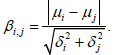Since the presence of outlier data is always possible with cytometry data, both methods were made more robust with quartile functions. The distance between cluster centers is defined as,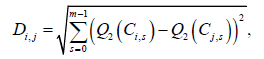Where,

m is the number of dimensions,

Q2 is the second quartile or median, and

Ci,s, Cj,s are the ith and jth cell type sets of the Sth dimension data.

The number of dimensions for the Cen-se′ and t-SNE maps is equal to two for this study. The minimum inter-distance for the Dunn index numerator is,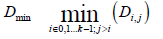The number of involved clusters is k=5 (CD8 T cells, CD4 T cells, B cells, NK cells, and monocytes). A reasonable measure of intra-distance is the average robust standard deviation, rSD, for all dimensions as defined by the first and third quartiles,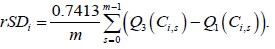The intra-distance between clusters i and j is given as,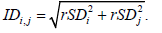The maximum intra-distance is,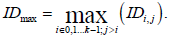The Dunn index is therefore computed as,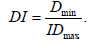The SSMD β value between cluster i and j is,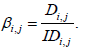The overall cluster index is given as,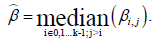Information loss (% IL)

The DKL value is a measure of information loss (Appendix) in units of nats for the mapping process. As described in the Appendix 1, a potentially more intuitive measure of information loss is the relative loss of information compared to the original information content or negative entropy of P. The percentage of information loss (% IL) is calculated as,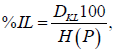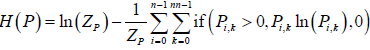The if function returns the second argument if the first argument is true and the third argument if it is false. As described in the Appendix 1, ZP is the sum of all high-dimensional similarities, P The number of events in the mapping process is ‘n’ and the number of nearest neighbors for each event is ‘nn’.

Cen-se′ mapping control properties

Pressing the <Alt> key while entering the Cen-se′ editor for Pathsetter exposes a rich set of properties that allow control of most parameters that guide the mapping system. These properties can be edited to duplicate most of the results shown. Table 1 summarizes these properties, their abbreviations, and their default values. Property values were in their default condition unless specified otherwise. Most experiments can be reproduced by changing these parameter values.

#### Results

Example data

A nine-point example will be presented and each step of the Cense′ algorithm will be described with comments on how it differs from the associated t-SNE algorithm when appropriate. The processing steps for the Cen-se′ method are shown in Figure 1. Details associated with each step are described below.

Figure 1: Cen-se′ processing. The processing of both t-SNE and Cen-se′ can be divided into phases. The Cen-se′ mapping system adds a step at the end to rapidly classify all events in an FCS file.

Step 1: Data transformations: M-dimensional measurement data are normally transformed to stabilize population variances [15,20- 23]. However, this nine-point example is designed to be as simple as possible and therefore has not been subjected to these transformations. The X′ rows, 0<=i<n, are considered “events” and the columns, 0<=j<M, are the correlated measurement values associated with the events. Note that the first three events have similar measurement values, as do the next three and final three. The Ids vector is not important for any of the following algorithms and is only included to help with algorithm descriptions.

Step 2: Scaling: The original t-SNE algorithm scales the data such that the means of each column of data are zero and the maximum absolute deviation from the means is either positive or negative unity. The Cen-se′ algorithm slightly modifies this approach by using medians instead of means and finding the maximum robust standard deviation, 0.741*IQR , from all M measurements and then dividing each element by that value. The Cen-se′ scaled data are shown below

Step 3: Nearest neighbor distances and vantage point tree: The t-SNE algorithm uses the free parameter, perplexity, to set the nearest neighborhood size, nn, to three times its value. The Cen-se′ method uses the free parameter, percent nearest neighborhood (% nn), to set the nearest neighborhood size. In this example, the nearest neighborhood size will be arbitrarily set to six, given the example’s very small size. Both algorithms then compute the nearest neighbor Euclidean distance matrix, D, and its associated event ID matrix by leveraging a vantage point tree algorithm .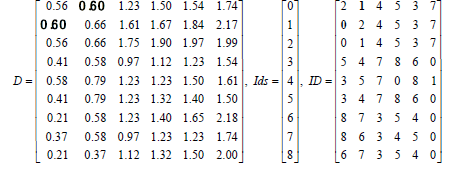Notice that the distance between event 0 and event 1, its secondnearest neighbor, is 0.60 (first row in D, bold), which is symmetric with the distance between event 1 and its nearest neighbor, event 0, (second row in D, bold).

Step 4: Pairwise similarities: The next step for both algorithms is to convert the nearest neighbor distances to probabilities, better described as pairwise similarities. The t-SNE algorithm assumes that each event is surrounded by a Gaussian-distributed probability kernel that has the general form,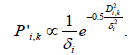If δi is known, then a proportional probability value,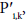can be calculated for each event. Since perplexity, the free parameter of the t-SNE method, is defined as the exponent of entropy (see Appendix, Information Theory Primer), entropy is calculated as,

H(P)=ln (perplexity).

Entropy  evaluated over the nearest neighborhood is defined in this context as,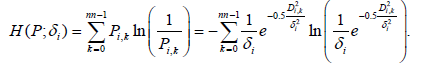The t-SNE method assumes that entropy is constant and therefore δi can be estimated for each event by an iterative divide-and-conquer solution.

The Cen-se′ method uses the Cauchy distribution  for both its high-dimensional and low-dimensional similarities and does not use either perplexity or entropy in any of its calculations. The Cauchy probability distribution, also known as the Lorentz distribution, is formally defined as,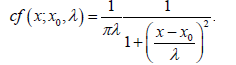In both t-SNE and Cen-se′ the offset, x0, and scaling parameters, λ, are set to 0 and 1 respectively and the probability density function’s inverse pi normalization factor is omitted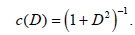The high-dimensional similarities are computed as,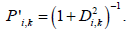Both methods perform a summation of the nearest neighbor similarities for each ith event.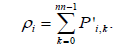By default, both methods then normalize each row of the similarity matrix to stabilize density-dependent differences (Figure 2).

Figure 2: Template mode and other maps. Panel A: 10,000 events were rendered in 38 seconds with the %nearest neighbor parameter set to 2. Panel B: After the initial mapping, 91,939 events were placed on the map by the Template Mode algorithm in 1.2 seconds. Panel C: Same as B but with measurements scaled with zero median and unit rSD. Panel D: Same as B but with no row normalization in step 4.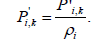This row normalization step results in cleaner-looking maps (Figure 2B for row-normalized map and Figure 2D for unnormalized row). The row normalization is performed on the simple example and the P′ similarities are,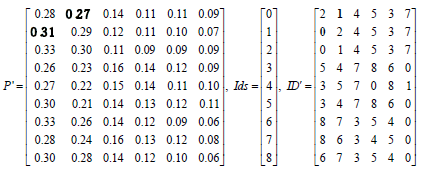Note that each row initially sums to unity due to row normalization; however, the P′ matrix is no longer guaranteed to be symmetric (bolded example P′ elements).

Step 5: Symmetrizing: Two types of asymmetries in the P′ matrix are important to rectify to obtain good mappings. If two events have different similarities (event 0 and 1 above), the similarities are set to the average of the two.

The other more serious asymmetry is if event i has event k in its neighborhood but does not have event i in event k’s neighborhood. The t-SNE algorithm uses an additive element method for ensuring this symmetry is maintained. In our example, note that event 1 has event 7 in its neighborhood, whereas event 7 does not have event 1 in its neighborhood. Why do we need to create a symmetric similarity matrix? The derivation of the gradient equations (Appendix) take advantage of this similarity property and dramatically reduce the number of required calculations by assuming that each element in a specific matrix row of the similarities has identical similarities found in other matrix rows.

The t-SNE algorithm will add event 1 to event 7’s neighborhood to create symmetry and therefore needs to maintain a variable row size type of matrix for large matrices, this process inflates the matrix size and complicates the format of the P matrix.

Cen-se′ uses a specially designed P′ matrix unpacking and packing algorithm to ensure that neighborhood symmetries are maintained (Appendix and Figure 3) and that the size of the P′ matrix remains unchanged. Elements of the matrix that are no longer used are flagged with an ID element value of –1 After the data are symmetrized with the Cen-se′ algorithm, the example matrices are:

Figure 3: Cen-se′ unpacking and packing of similarities. Point-pair similarities are pushed onto a priority queue and sorted in probability order when all point pairs are unpacked and put onto the priority queue, they can be popped off and packed into the similarity matrix.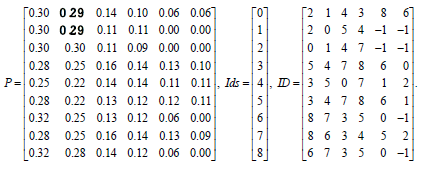Notice that the P matrix now has symmetric similarities and the ID matrix has different sets of elements due to the unpacking and packing process. The event 0-1 pair and the event 1-0 pair have the same similarity of 0.29 (bold).

Another difference between the two algorithms is that Cen-se′ does not normalize all elements of P such that they add to unity, which prevents serious round-off errors with large matrices (Step 4 Discussion for other advantages). Instead, it saves the summation of all similarities as a double-precision Zp variable,

Another difference between the two algorithms is that Cen-se′ does not normalize all elements of P such that they add to unity, which prevents serious round-off errors with large matrices (Step 4 Discussion for other advantages). Instead, it saves the summation of all similarities as a double-precision Zp variable,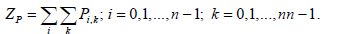and uses it in the gradient equations (Step 9 and Appendix for details).

Step 6: Y initialization: Each of the n X high-dimensional points has an associated low-dimensional point, Y. The dimensionality of Y is given by m, which is normally set to two (m<<M). If Cen-se′ and t-SNE are in a stand-alone mode, they both will initialize the Y points into a very small spot (sd<0.0001) using a normally distributed random number generator.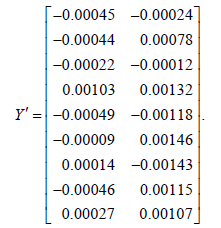The SD of the initial spot size for t-SNE and Cen-se′ is 0.0001. See the Results Experiment 4 for details and Experiment 6 for what happens if Cen-se′ is coupled to probability state modeling [28-31]. At this point, the algorithm enters a repetitive sequence of operations where most of the processing is done (Figure 1, Steps 7-9).

Loop step 7: Y Normalization: Both t-SNE and Cen-se′ normalize each dimension such that its mean is zero. The initial sample Y points are,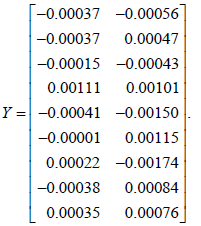Neither t-SNE nor Cen-se′ stores the low-dimensional distances or similarities.

Loop step 8: P matrix α multiplier: Both the t-SNE and Cen-se′ algorithms initially increase the similarities in the P matrix by a factor, α, of 12. The Cen-se′ method uses the factor in its gradient equation (Step 9), whereas the t-SNE method directly modifies the P matrix elements. This step is discussed in more detail later in the Experiment 5 Results and Discussion sections.

Loop step 9: New Y values: A quick primer on information theory and the derivation of the gradient equations are in the Appendix. Both t-SNE and Cen-se′ have equivalent gradient equations; however, they are posed differently. The Cen-se′ gradient equation for the ith point and sth dimension is derived in the Appendix and is given as,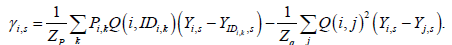The left side is computed over the k nearest-neighborhood domain, whereas the right side is computed over all j point pairs. Because of the enormous number of i-j interactions for estimating Zq and the gradient point, typical maps with 105 to 107 events use a Barnes-Hut algorithm  to approximate this term. Once both terms of the gradient equation are computed, the change in the ith Y point’s s dimension is given as,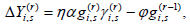Where,

r is the iteration number,

η is a constant,

α is an attraction factor,

ϕ is the momentum factor.

More details are available in the Appendix section. The Cen-se′ algorithm temporarily increases point-pair attractions by increasing α to 12, whereas the t-SNE algorithm modifies the elements of P directly. After the Y points are updated, both algorithms loop back to Step 7 (Figure 1 for overview). By default, the t-SNE method iterates 2,000 times whereas the Cen-se′ method only iterates 1,000 times to minimize DKL.

Figure 4 shows the nine-point DKL Cen-se′ response function (red) and slope or gradient lines (solid blue and dashed green) for a set of new Y values ranging from -1 to 1 for one of the nine points. The solid blue line represents a brute force slope determination about each new Y value and the superimposed dashed green line is the derived gradient equation value. Their coincidence supports the veracity of the assumptions made in the gradient’s derivation. The vertical black line is the initial location of the point, which is near zero, and the horizontal black line is the zero-gradient line. The gradient for the initial value is calculated as positive (black circle above the horizontal black line) for this point, which means the trajectory for this point’s dimension will be to decrease its value (see arrow).

Figure 4: DKL and gradients. The DKL Response function (red) and slope or gradient lines (solid blue and dashed green) for new Y values. The solid blue line represents a brute force slope determination about each new Y value and the superimposed dashed green line is the derived gradient equation value. The vertical black line is the initial location of the point that is near zero and the horizontal black line is the zero-gradient line. The gradient for the initial value is calculated as positive (above the horizontal black line) for this point, which means the trajectory for this point’s dimension will be to decrease its value (see arrow).

There can be multiple local DKL minimums as shown above for both Cen-se′ and t‑SNE, but they don’t seem to pose a problem for either this simple example or complex cytometry data (Discussion for more details). The rate of DKL minimization is shown in Figure 5. After a brief expansion phase where points rapidly move away from each other, the DKL quickly minimizes to a value of 0.141 for Cen-se′ mapping. In this simple example, α did not change from unity.

Figure 5: DKL minimization. The initial randomly distributed Y points had a DKL value of 0 517. After 50 iterations, the DKL value minimized to 0 141. The inset shows the point trajectories as the iterations take place. The points expand out of the center during the expansion phase and their trajectories are shown with small black circles in the inset graph. Before minimization the correlation between the P and Q similarities was 0.00275 and after minimization it was 0.946.

The Figure 5 inset shows the nonlinear trajectories for each of the nine points during the minimization process. The first three adjacent points end up being grouped together (red circles) along with the second three (blue squares) and last three (green triangles). Before the minimization starts, the correlation between the high and low similarities was 0.003 and after minimization, it was 0.95.

Steps 1 through 9 have taken three-dimensional data with three sets of clusters and positioned the corresponding two-dimensional points such that their pairwise similarities are highly correlated. Whether it is three dimensions or 30, both Cen-se′ and t-SNE algorithms move lowdimensional points in highly nonlinear ways to best represent highdimensional sets of similarities. But why didn’t the DKL value minimize to a near-zero value?

The answer is better-understood if the high-dimensional P and two-dimensional Q similarity matrices are properly reposed for this nine-point example.

The P matrix has been extended by two columns to include all eight nearest neighbor points. The assumption that is tacitly made in the theory is that P similarities beyond an event’s nearest neighborhood are zero. By dividing by their respective totals, the two matrices become probability matrices that can be directly compared. First note how similar the first three columns are for both matrices. These points represent the relatively high similarities for the three clusters of threepoint data. It is this correspondence that is responsible for the mapping routine working as well as it does. Note that those P elements with zero values have non-zero values for corresponding Q probabilities. This lack of correspondence for zero P elements is responsible for DKL not approaching zero with higher iterations. Another consequence of this lack of correspondence is that in order to make those elements approach zero, the map must constantly expand.

A perfect solution for the nine-point example would be to set the number of nearest neighbors for each event to eight. In this configuration, all P similarities would have non‑zero probability values Figure 6 compares the nn=6 to the perfect nn=8 solution for DKL minimization (left-hand axis) and the expansion of points (right-hand axis). Expansion is defined in this context as the maximum distance between any two points. If all point pairs are accounted for, DKL values approach zero and the expansion approaches a constant value of 2.059 with iteration number. Perfect solutions like this are not normally feasible with typical mappings and SNE implementations, but this simple nine-point example helps explain some commonly observed features of the SNE mapping process.

Figure 6: Nearest neighbor vs. perfect solution. Left axis plots compare the nearest neighbor, nn=6, to the “perfect”, nn=8, solution for the nine-point DKL minimization. The perfect solution minimum approaches zero, whereas the nearest neighbor solution does not. The right axis plots compare the expansion of the maps. The perfect solution approaches a constant level of expansion, whereas the nearest neighbor solution continues to expand with iteration.

Step 10: Template mode: In this simple example, all points are used to develop the map; however, for typical cytometry data, a subset of points is usually selected. By default, Cen-se′ samples 10,000 events from a FCS3.0 file with a % nn set to 2%, which creates 200 nearest neighbors for each event. The free parameter, % nn, is editable, but it is rarely necessary to change it.

Once the map is complete, the vantage point tree can be leveraged to locate all points in the file to nearest neighbor points in the map. The points are placed on the map with a small normally distributed dither. Typically, the speed of this process for typical PCs is approximately 100,000 events per second, which means that even files with millions of events can all be represented on the map in less than a minute. Figure 2 shows some 30-dimensional mass cytometry data before (Panel A) and after (Panel B) Template Mode is applied to the data. Template Mode rendered the 91,939 events (excluding granulocytes) that were in the file in 1.2 seconds. The other two panels show maps from data where the measurements have unit standard deviation (Panel C) and were not row-normalized (Panel D). These two panels are discussed more in the Discussion section.

Experiments

Cen-se′ mapping parameters and their abbreviation are shown in Table 1.

Label Default Cen-se' Mapping Properties
Description
ni 1000 Number of iterations
n 10000 Number of events to map
%nn 2 Percentage of n for nearest neighborhood size
nn 200 Number of nearest neighbors
pp 50 Perplexity, free parameter for t-SNE
h ln(pp) Entropy, ln(pp)
nd No Normal distribution, Yes for TSNE, No for Cen-se'
ri No Random initialization, No for using summary map
rs 1079 Random seed if ri=Yes
lc Yes Log Cauchy
iss 0.0001 Initial spot size
α 12 Initial attraction factor
φ 0 Initial momentum factor

Table 1: Cen-se′ mapping properties all properties shown are available if key is held while double-clicking to obtain the Cen-se′ editor.

Experiment 1: t-SNE and Cen-se′ comparisons: Three different numbers of mapping events were tested: n=10,000, 50,000, 100,000; for the t-SNE and Cen-se′ methods, nd=Yes (t-SNE), No (Cen-se’), lc=No; with random initialization, ri=Yes, rs=1079 for File 1. The leftmost panels in Figure 7 represent t-SNE maps with 10,000; 50,000; and 100,000 events. The right-most panels represent the corresponding Cen-se′ maps. The top-center panel compares the loss of information, DKL , for t-SNE and Cen-se′. t-SNE loses more information in the mapping process with increasing numbers of events, whereas Cen-se′. loses less information and is constant for increasing number of events The middle-center panel compares the Dunn index (DI), a worsecase metric for cluster separation. The t-SNE algorithm loses cluster separation with higher numbers of events, whereas Cen-se′ does not. The lower-center panel compares the clustering index (CI), a central tendency type of metric, for cluster separation. The same patterns are observed as for the Dunn index.

Figure 7: t-SNE and Cen-se′ comparison. The left-most panels represent t-SNE maps with 10,000, 50,000, and 100,000 events The right-most panels represent the corresponding Cen-se′ maps. The top-center panel compares the loss of information, DKL, for t-SNE and Cen-se′. t-SNE loses more information with increasing numbers of events. The middle-center panel compares the Dunn index, a well-known worse-case metric for cluster separation. The t-SNE algorithm loses cluster separation with higher events, whereas Cen-se′ does not. The lower-center panel compares the clustering index, a central tendency type of metric, for cluster separation. The same pattern is observed as for the Dunn Index.

Experiment 2: t-SNE and Cen-se′ more comprehensive comparisons: A more comprehensive comparison between the two methods was tested. The comparison includes an addition file, random seed, and t-SNE perplexity values. A summary of the results is shown in Table 2. Even with higher perplexity values, the Cen-se′ method always has less loss of information (DKL and %IL) and higher cluster separation (CI and DI) than corresponding t-SNE maps.

Experiment 2: t-SNE  and Cen-se′ More Comprehensive Comparisons
Method Number %nn/Perplexity nn File Rnd DKL %IL CI (β) DI
Cen-se' 10000 1.5 150 1 1 1.36 9.61 4.95 2.33
50000 1.5 750 1 1 1.37 7.90 5.39 2.83
100000 1.5 1500 1 1 1.36 7.26 5.50 2.79
t-SNE 10000 50 150 1 1 1.59 11.91 3.51 1.27
50000 50 150 1 1 2.67 17.93 2.69 1.16
100000 50 150 1 1 3.21 20.52 2.51 0.77
50000 250 750 1 1 1.67 10.14 3.55 1.87
100000 500 1500 1 1 1.68 9.41 3.83 1.57
Cen-se' 10000 1.5 150 1 2 1.36 9.61 6.45 2.73
50000 1.5 750 1 2 1.37 7.87 5.48 2.59
100000 1.5 1500 1 2 1.36 7.27 5.74 2.35
t-SNE 10000 50 150 1 2 1.57 11.82 3.54 2.20
50000 50 150 1 2 2.69 18.00 3.26 1.29
100000 50 150 1 2 3.20 20.50 2.30 0.65
50000 250 750 1 2 1.67 10.13 3.40 1.50
100000 500 1500 1 2 1.68 9.37 3.86 1.57
Cen-se' 10000 1.5 150 2 1 1.55 10.96 6.04 2.80
50000 1.5 750 2 1 1.57 9.08 4.72 2.78
100000 1.5 1500 2 1 1.58 8.41 5.68 3.16
t-SNE 10000 50 150 2 1 1.81 13.61 3.85 2.16
50000 50 150 2 1 2.93 19.61 2.69 1.64
100000 50 150 2 1 3.47 22.19 2.35 1.05
50000 250 750 2 1 1.92 11.62 3.34 2.24
100000 500 1500 2 1 1.93 10.79 4.35 2.19
Cen-se' 10000 1.5 150 2 2 1.55 10.98 4.97 2.24
50000 1.5 750 2 2 1.57 9.05 5.12 2.81
100000 1.5 1500 2 2 1.57 8.38 5.31 2.58
t-SNE 10000 50 150 2 2 1.82 13.64 4.36 2.07
50000 50 150 2 2 2.93 19.61 3.15 1.46
100000 50 150 2 2 3.46 22.16 2.73 1.25
50000 250 750 2 2 1.94 11.70 3.81 2.12
100000 500 1500 2 2 1.94 10.82 4.34 2.30

Table 2: Experiment 2 t-SNE and Cen-se′ More Comprehensive Comparisons Different numbers of mapping events, nearest neighborhood sizes, files, and random numbers were tested for Cen-se′ and t-SNE mappings DKL is the loss of information in units of nats, %IL is the percent information loss, CI (β) is the cluster index, and DI is the Dunn index Better maps have lower DKL and %IL values and higher CI and DI values In all cases, Cen-se′ Cauchy distributed high-dimensional similarities outperformed the corresponding t-SNE normal distribution similarities.

Experiment 3: t-SNE and Cen-se′ random data comparison: Another approach to comparing the two methods is to modify the program to use 30‑dimensional uniform random data [rnd(1)*100] and then create a perfect map, n=1,000 and nn=999, for both t-SNE and Cen-se′. The results of this experiment are a DKL and %IL of 2.31 and 21.04 for t-SNE and 0.02 and 0.17 respectively for Cen-se′. The results are that the Cen-se′ method loses at least 100 times less information when mapping high-dimensional random data to two dimensions.

Experiment 4: Optimal initial spot Size for Cen-se′: For this experiment File 1 was the source of data with ni=2000, α=1.0, ri=Yes, rs=1709. A range of initial spot sizes were examined: iss=0.001, 0.01, 0.1, 1.0, 10.0; and the %information lost tabulated as a function of iteration (Figure 8). All values of initial spot size ultimately resulted in less than 10% information lost; however, the higher spot sizes required more iterations to minimize DKL . File 2 showed the same pattern (data not shown).

Figure 8: Optimal spot size. Different initial spot sizes were examined for File 1 with ni=2000, α=1.0, ri=Yes, rs=1709; iss=0.001, 0.01, 0.1, 1.0, 10.0; and the %information lost tabulated as a function of iteration. All values of initial spot size ultimately resulted in less than 10% information lost; however, the higher spot sizes required more iterations to minimize DKL.

Experiment 5: Optimal α and ϕ factors for Cen-se′ and t-SNE: This experiment examined the set of α factors 1, 2, 4, 8, 10, 12 and momentum factors ϕ=0.0, 0.5, for File 1 and 2 with ni=2000, ri=Yes, rc=1709, iss=0.0001, and lc=No for the Cense′ and t-SNE methods. The Cen-se′ method used Cauchy distributions (nd=No) for creating high-dimensional point-pair similarities and the t-SNE method used normal distributions (nd=Yes). The loss of information parameters, DKL and %IL, and clustering indices, CI and DI, were evaluated for each map Maps with relatively low DKL and %IL and high CI and DI are considered desirable. The results are summarized in Table 3. For the Cen-se′ method, any α factor>1 was satisfactory and the momentum factor, ϕ=0.5, did not improve the maps appreciably. The t-SNE method may have a modest optimum value of α=8 and ϕ=0.5.

Experiment 5: Optimal α and ϕ Factors for Cen-se′ and t-SNE
ni=2000, ri=Yes, rc=1709,iss=0.0001, Ic=No
nd α φ File DKL %IL CI DI
No 1 0.0 1 1.44 9.99 5.00 2.27
No 2 0.0 1 1.20 8.30 6.32 3.13
No 4 0.0 1 1.20 8.30 5.97 2.54
No 8 0.0 1 1.20 8.30 6.60 2.72
No 10 0.0 1 1.20 8.33 6.78 2.80
No 12 0.0 1 1.20 8.32 6.61 2.91
No 12 0.5 1 1.20 8.32 6.18 2.74
No 1 0.0 2 1.63 11.30 7.28 3.51
No 2 0.0 2 1.41 9.76 6.20 2.36
No 4 0.0 2 1.40 9.75 5.37 3.21
No 8 0.0 2 1.41 9.77 5.34 2.98
No 10 0.0 2 1.41 9.76 5.55 3.26
No 12 0.0 2 1.41 9.76 5.52 3.42
No 12 0.5 2 1.41 9.78 5.73 3.08
Yes 1 0.0 1 1.69 12.67 1.45 0.40
Yes 2 0.0 1 1.57 11.77 2.77 0.93
Yes 4 0.0 1 1.52 11.39 3.44 1.77
Yes 8 0.0 1 1.51 11.32 3.66 1.95
Yes 10 0.0 1 1.51 11.33 3.47 1.79
Yes 12 0.0 1 1.52 11.37 2.95 1.45
Yes 12 0.5 1 1.52 11.44 3.38 1.96
Yes 1 0.0 2 1.88 14.04 2.59 0.98
Yes 2 0.0 2 1.77 13.25 3.20 1.50
Yes 4 0.0 2 1.73 12.96 3.35 1.95
Yes 8 0.0 2 1.77 13.23 3.57 1.91
Yes 10 0.0 2 1.74 13.06 3.45 1.85
Yes 12 0.0 2 1.78 13.34 3.14 1.83
Yes 12 0.5 2 1.70 12.72 3.99 2.14

Table 3: Experiment 5 A set of α: 1, 2, 4, 8, 10, 12, and ϕ; 0.0,0.5 factors were examined for File 1 and 2 with ni=2000, ri=Yes, rc=1709, iss=0.0001, and lc=No The top rows of the table use the Cauchy distribution (Cen-se′, nd=No) for high-dimensional similarities and the bottom rows use a normal distribution (t-SNE, nd=Yes) For the Cen-se′ method, any α factor>1 was satisfactory and the momentum factor, ϕ=0.5, did not improve the maps appreciably The t-SNE method may have a modest optimum value of α=8 and ϕ=0.5.

Experiment 6: Structured cell type seeding for Cen-se′: The SNE algorithms are inherently stochastic and tend to put populations or clusters in random positions. Figure 9, left panels shows four Cen-se′ maps with different initializing random number seed values (ri=Yes, rs=1709, 1708, 1707, and 1706) for File 1. These maps show how populations change their location and orientation with different initial random event positions.

Figure 9: Structured cell type seeding. The left Cen-se′ panels show how populations change their positions and orientations with different random number sequences starting at different seed values (1709, 1708, 1707, and 1706). The middle panel shows a probability state modeling summary map of all enumerated populations. If instead of a single spot, a scaled-down summary map is used to initialize event positions, the final position and orientation of the cell type events remain relatively consistent.

Each of these maps randomly positions events into a single spot determined by the initial spot size parameter. If instead of a single spot, a more structured cell type seeding is employed, the final relative location and orientation of the cell type clusters remains more consistent from map to map (right panels). In this case, the PSM summary map (center map) guided the initial seeding process.

#### Discussion

Example data

Step 1: Data transformations: In general, cytometry linear measurements should be transformed by a log-like transform. Very poor-resolution maps result from using linear cytometry data (results not shown). A few implementations of the t-SNE algorithm use the hyperbolic arcsine function to convert linear data that may have negative signed numbers to log-like distributions. If the simpler transform is all that is available, it is very important to examine each one-dimensional measurement histogram to ensure that the transform is not artificially splitting low-intensity populations into two separate populations. If possible use well-established transforms like Logicle [21,23], HyperLog , or VLog  to stabilize population variances for cytometry-derived data. If possible, consider normalizing the data to minimize undesirable batch effects .

Step 2: Scaling: The t-SNE method uses means and maximum absolute deviations from the mean to scale the data prior to mapping. Since cytometry data normally have outliers, Cen-se′ uses medians instead of means and a maximum robust standard deviation instead of the maximum deviation to scale data prior to mapping. Statisticians may wonder why measurements are not normally converted to standardized variables with unit standard deviations. Maintaining the variance for each measurement generally produces better-looking maps than fully standardized maps. Figure 2C shows an example of a suboptimal map that uses standardized data.

Step 3: Nearest neighbor distances and Step 4: Pairwise similarities: The t-SNE method’s use of perplexity to determine the nearest neighborhood size, nn, as well as computing the kernel standard deviations, δi, is one of its major shortcomings. There are at least three major issues with this strategy. The first issue is that assuming entropy is constant is not tenable, especially when dealing with biological systems. The second is that by binding the nearest neighborhood size and the event’s kernel standard deviation through perplexity, the t-SNE algorithm cannot be properly optimized for data such as generated by Cytometry. The third is that there is no reason why the underlying probability distributions for generating similarities should be inconsistent for high-dimensional and low-dimensional data. By using a normal distribution for high dimensions and Cauchy for low dimensions, the t-SNE mapping efficiency is significantly reduced (Experiment 3 for a “perfect” example of this).

If t-SNE methods are modified so that nearest neighborhood size and entropy are separate free parameters, then the t-SNE method can be optimized to give resolutions similar to those of Cen-se′ (data not shown). However, given that the Cen-se′ method is far simpler to compute and uses only one easy-to-understand free parameter, there is no advantage to doing this.

Both Cen-se′ and t-SNE methods normalize each row of similarities to unity. This is an important step for producing maps with wellseparated clusters. Figure 2D shows the deterioration of the map if the row normalization step is skipped.

Cen-se′ does not normalize all the similarities to unity to form probability values. Instead, it computes a double-precision summation value, ZP, and uses that in its gradient equations. At first this seems like a relatively minor enhancement, but it ends up allowing the implementor to encode the similarity matrix as single-precision floats instead of double-precision floats. The impact of this relatively simple change is that the t-SNE matrices are at least twice the size of equivalent Cen-se′ matrices. These matrices can be quite large and saving this space can often prevent running out of cpu memory. The other advantage of having the P matrix as single-precision floats is that it lends itself to massively parallel GPU solutions.

Step 4: Other distance to probability functions: Anecdotal evidence suggests that the Log-Cauchy distribution may be slightly better in discriminating small populations than Cauchy. The Log- Cauchy option for Cen-se′ is given as,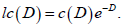Another variant of the Cauchy distribution that was explored is the Cauchy with its scale parameter, λ,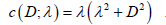Somewhat surprisingly, neither of the above functions appreciably changed the information loss or clustering index of the Cen-se′ maps (data not shown).

Step 5: Symmetrizing: The method that t-SNE uses to symmetrize its similarity matrix is another weak area in the algorithm. The t-SNE algorithm has variable row size matrices in order to add events to rows that do not have symmetric events. The result of this process is that matrices have approximately 30% more elements than matrices with constant row size of nn. The extra elements not only slow down the new Y calculations (data not shown) but also make an already large matrix bigger, often resulting in program crashes.

The unpacking and packing process described in the Appendix results in matrices that have nn row size. The percentage of elements flagged as not used is usually reasonably low, ranging between 6% and 7% of the total number of elements. For example, File 1 with n=10,000 and nn=200, was 6.08% and File 2, 6.98%

Steps 6, 7, 8, and 9: These steps are discussed further in the Experiments section.

Step 10: Template mode: Once the Cen-se′ map is rendered, it can be leveraged to quickly categorize all the events in the file. The vantage point tree can be interrogated to find a nearest neighbor map point Once it finds this point, it can be displayed on the map by placing it in a slightly dithered location from its nearest neighbor point. Typically, the dithering is done by a normal distribution random number generator with its SD set to 0.3. The speed of this process for typical PCs is around 100,000 events per second.

This ability has far-reaching possibilities for cell sorters. Maps can be rendered, and populations identified by analysis packages like GemStone. Users can then create regions around populations defined by many dimensions and sort these populations for further study.

Experiments

Experiments 1-3: Cen-se′ and t-SNE: These experiments show that Cen-se′ has a strong tendency to outperform t-SNE for minimizing the loss of information and separating cell type clusters for a wide range of conditions. There are probably many factors for this improved performance, but the major factor is the consistent distance to probability function for both high-dimensional and low-dimensional similarities. In many ways, Experiment 3 is the most convincing of the three. If 30-dimensional uniform random data are mapped to two dimensions, the Cen-se′ method loses less than 0.2%, whereas t-SNE loses more than 20%. Mapping a uniform set of events is a worse-case scenario since virtually all combinations of measurement values are represented in the mapping.

Experiment 4: Optimal initial spot size for Cen-se’: The smaller the initial spot size, the closer the events are in the beginning of the mapping process. Even with relatively large spot sizes, the map ultimately minimizes the information lost, DKL . Our interpretation of these results is that the minimization process is most efficient when events are forced to be very close to each other. When events are highly concentrated, gradient forces are highest and points quickly move to optimal locations in the map. Although this size could easily be a range, the default is set to 0.0001 to correspond with t-SNE.

Experiment 5: Optimal α and ϕ factors for Cen-se′ and t-SNE: The α factor is another mechanism to concentrate events to optimize DKL minimization. If α is significantly greater than 1, the increased attraction gradient force causes events to concentrate much like initial spot size. The two parameters work synergistically to make sure the similar events come into close proximity. Cen-se′ resets α to 1 at iteration 200 while t-SNE resets at 250. The data shown in Table 3 suggests that Cen-se′ is not affected much by α. Even though no clear optimal value was found with the data tested, a default of 12 is used in Cen-se′ to correspond with t-SNE just in case other datasets would benefit from this additional temporary attractive force.

Both t-SNE and Cen-se′ have a momentum term, ϕ, in the gradient equations. The idea behind this term was that it might help avoid suboptimal solutions that were due to points trapped in local minimums as seen in Figure 4 (New Y Value~0.3). Our current hypothesis as to why this does not seem to happen is that local minimums probably only appear transiently, especially in the early stages of the optimization. As soon as the other points change locations in subsequent iterations, the local minimum probably disappears, allowing the stalled event to move again

#### Conclusion

The ability to visualize high-dimensional data in low dimensions with minimal loss of information has many applications. The highlevel conclusion to make from the theory and experiments presented is that if the same type of function is used to create both high- and lowdimensional similarities, then mapping efficiency can be maximized. Minimizing the loss of information in this mapping process has great value for better understanding any data that is encoded in high dimensions The last step in this process, called “template mode” in Cen-se′, has the potential of revolutionizing how specific cells can be identified, sorted, and studied.

#### Acknowledgements

This work could not be accomplished without the help of all the employees of Verity Software House. Thanks goes out to the editors at Fluidigm Corporation for wading through this manuscript and making numerous improvements. Finally, we have to acknowledge that the wide use of many effective dimensionality reduction methods can be traced back to van der Maaten and Hinton for making their algorithms available in multiple languages on web sites.

#### Disclosures

GS is an employee of, and receives remuneration from, Fluidigm Corporation. Fluidigm, Helios, Maxpar and Pathsetter are trademarks and/or registered trademarks of Fluidigm Corporation in the United States and/or other countries. All other trademarks are the sole property of their respective owners. For Research Use Only Not for use in diagnostic procedures.

#### Conflict of Interest

The authors Bagwell, Bray, Herbert, Hill, and Hunsberger all work for Verity Software House that markets software that contains Cen-se’ mapping. Inokuma is a consultant for Verity Software House and Stelzer works for Fluidigm Corp that markets and sells this software product.

#### Appendix

Unpacking and Packing for Symmetrizing

The Cen-se′ method for symmetrizing similarity matrices is fundamentally different than the t-SNE method. The algorithm begins with the first event, index 1, and its nearest neighbor (index 2). It looks at its nearest neighbor row to see if it finds its index (index 1) listed. If the nearest neighborhood contains its index, it creates a C++ object that contains the average similarity value for the two point pairs, the first index, index 1, and the second index, index 2. If it does not find its index in the neighborhood, it creates an object with the two indices and records only the single similarity value.

The key to the algorithm is to push this object onto a priority queue. After all the nine-point example point pairs have been pushed onto the priority queue, it has the structure shown in Figure 9. All the objects containing the probability, index 1, and index 2 values are sorted in decreasing probability order. The routine then starts popping the objects off the queue and putting them into the P similarity matrix if there is room to put in both point pairs. At the end, it flags those elements not packed with similarities values of -1.

Information theory primer

The theory that underlies all these mapping routines is information theory. The best way of understanding this important theory is by means of simple examples. Suppose we have four boxes and there is a ball hidden in one: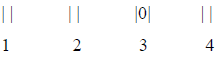There is an equal chance of the ball being in any one of the boxes, which means that the probability of its being in any one box is 1/4 or 0.25. Suppose you can only ask “yes” or “no” types of questions to find the ball. How would you go about finding it?

A rather mindless approach might be asking whether it is in box 1. If it is not there, “Is it in box 2?” might be asked. Proceeding in this manner, the chance of finding the ball with one question would be 1/4 and the chance finding it with more than one question would be ¾.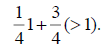The chance of picking the ball with the second question would be 3/4*1/3. If it wasn’t in this second choice, then the chance of finding the ball with the third question would be 3/4*2/3. The average number of yes/no questions to find the ball with this strategy would be 2.25 questions.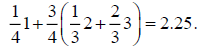A more optimal way of finding the ball with yes/no questions is to group the questions so that there is an equal chance of a “yes” or “no ” In this case, the questions might be,

Is the ball in box 1 or 2?

If “yes” then is it in box 1?

If “no” then is it in box 3?

For the four-box example, the minimum average number of “yes” or “no” questions would obviously be two. To generalize, if we let H(P) represent this minimum average, then the equation that would give us our answer for equal probability boxes would be,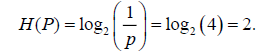If there were eight boxes, the minimum number would be three questions, and if 16 boxes, four. This simple formula always yields the correct answer if the probability of the ball in the box is equal for all boxes. Suppose that the probability of the ball being in a box varied and was given as,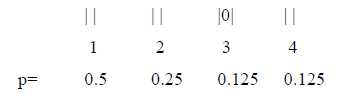With these probabilities, the questions that would have equal chance of a yes or no reply would need to be posed as,

Is it in box 1?

If “yes”, then we found it.

If “no”, is it in box 2?

If “yes” then we found it.

If “no” then is it in box 3?

If “yes” then we found it.

If “no” then we found it.

The minimum average number of questions is given as,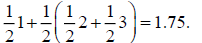In 1948, Shannon (26) discovered that this answer is more generally computed as,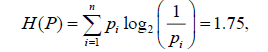and gave it the name entropy. Entropy is a measure of disorder and randomness and is used in signal transmission theory, thermodynamics, artificial intelligence, and many other technologies. If the number of boxes in this example is computed from entropy, the answer is called perplexity and it is the main free parameter of SNE and t-SNE but is not used at all in Cen-se′.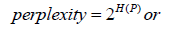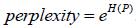If entropy is computed with the base 2 log, then the answer is in units of bits. If it is computed with the natural log, the answer is in nats.

Kullback-Leibler Divergence, DKL

Suppose that our real box probabilities were P: (0.5, 0.25, 0.125, 0.125) and that we didn’t know what the real probabilities were and guessed that they were Q: (0.25, 0.25, 0.25, 0.25). The entropy associated with P is 1.75 bits and the entropy associated with Q is 2 bits.

We might guess that the amount of information we would lose by approximating P with Q should be around 0.25 bits. A more accurate way of estimating this loss of information is the Kullback-Leibler divergence formula (33),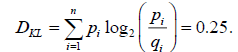The t-SNE and Cen-se′ methods use DKL as an objective function that is minimized by moving the lowdimensional data points, Y, along calculated trajectories. The percentage of information lost, %IL, by approximating P with Q is a useful metric for quantifying the progress of force-directed solutions to minimizing DKL,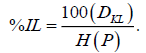To calculate what the Y trajectories should be, the natural log version of DKL is differentiated with respect to each dimension for each Y point.

Before jumping into our calculus calisthenics, let us first look at the problem from a high-level perspective. In a perfect world, all non-identical point pairs should be examined when calculating the high- and lowdimensionality similarity matrices. However, there are too many combinations of point pairs to examine with typical numbers of events ranging from 105 to 107. To make this problem solvable with widely available computer systems, a subset of P nearest neighbor point pairs is found and all point pairs outside these nearest neighbor regions are ignored. This approximation should make intuitive sense.

Along a row of the P similarity matrix, the similarities become smaller rapidly and it is therefore a reasonable proposition to ignore their small value after the nnth nearest neighbor. However, this simplifying proposition cannot be made with the low-dimensional point pairs. Because these points are randomly placed in low-dimensional space, their point-pair similarities are also initially random. Events that are nearest neighbors in high-dimensional space are usually not nearest neighbors in low-dimensional space.

Before beginning these derivations, there are a few definitions that will simplify the upcoming mathematics. Let i and j be integers that represent events i and j respectively. If S is the set of integers ranging from 0 to n-1, let i be an element in that set and j be an element that is not i. Initially let P(i,j) and Q(i,j) be defined as similarity functions instead of matrices and ignore for now the notion of nearest neighbor events.

Both P and Q similarities are guaranteed to be symmetric, that is,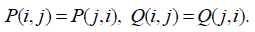Given these qualifications, the DKL objective function can be written as,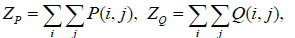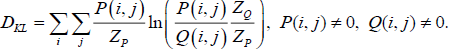Since,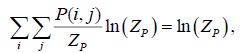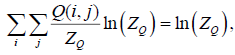The equation simplifies to,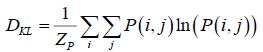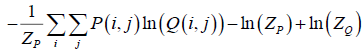The overall objective of the algorithm is to move the Y points to minimize DKL, which quantifies the information loss when approximating the P with Q similarities. To properly move Y point’s values, the derivative of DKL with respect to each dimension of each Y point must be calculated,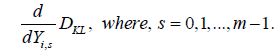If a Y point’s dimension value has a positive slope, then the desired trajectory for that point will be in the opposite direction, since the DKL function is to be minimized. The derivative equation can be simplified by observing what elements of the equation will not change if specific Y dimension values change. The first and third terms involving only P will not change when changing Y values and therefore their derivatives will be zero. Also, if Yi,s changes, then each element in the ith row for P and Q will change. Because each of these ith row elements have identical symmetric elements in other rows, only the ith row derivatives need evaluation. The summation of these derivatives only needs to be multiplied by two to be accurate. The derivative equation for calculating the slope of DKL with respect to Yi,s is,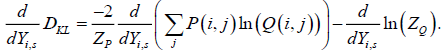Given that the derivative of ln(x) is 1/x dx, the above equation can be reposed as,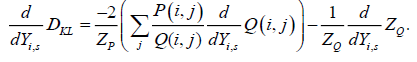The Q(i,j) derivative is,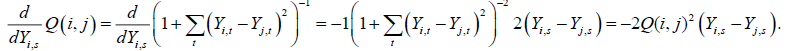Before evaluating the ZQ derivative, it is useful to first look at the ZQ summation and observe what elements change when Y i,s changes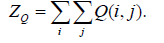When Y i,s changes, all the elements in the ith row of Q will change, but since each element in this row has an identical symmetric element in other rows, the derivative simplifies to,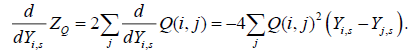The derivative equation for Cen-se′ is therefore,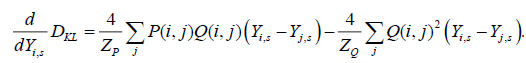Note, the t-SNE derivative equation does not have the ZP summation value to express the P similarities as probabilities. Although the t-SNE gradient formula is posed a bit differently (2), it is equivalent to the above formula. Because the P matrix defines just the nearest neighbor similarities, the P Q i-j cross product can be reposed using nearest neighbor similarities as,Also,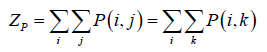Since the gradient, γ, only needs to be proportional to the derivative, its final form can omit the constants. It is convenient to split the gradient into two balancing parts. The part that tends to attract event pairs is,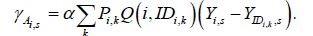The α factor is used to temporarily increase the attractive force between event pairs during the mapping process. In t-SNE and Cen-se′ it is set to 12 for the first part of the iteration cycle and then set to 1. The t-SNE method modifies the P matrix elements directly by multiplying by α and then later dividing by α. This artificial and temporary enhancement to the attraction part of the gradient has some desirable properties, especially for t-SNE, and will be examined in more detail in the results section, Experiment 5.

The matrix, ID, is used to find the appropriate kth nearest neighbor event. Since the attractive part of the gradient is computed over the nearest-neighbor domain, it tends to be computationally efficient. The gradient part that tends to repulse event pairs is,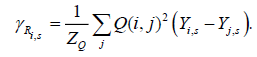Because of the large number of calculations necessary for the repulsive force component of the gradient, an approximation using a space tree is often desirable (32). Both Cen-se′ and t-SNE use 0.5 for the Barnes-Hut space tree omega parameter. In Cen-se′, both force computations are highly threaded for maximum performance. The overall gradient for a change to Y i,s is,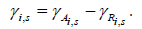New Y Values

The new Y values, Y(r), for the rth iteration could be computed directly from the gradient as,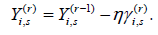where η is some proportionality constant. However, both t-SNE and Cen-se′ are more sophisticated in how they calculated new Y values to enhance the rate of convergence. For the first iteration, both methods initialize an adaptive gain and previous delta Y matrix as,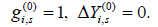The adaptive gain function is defined as,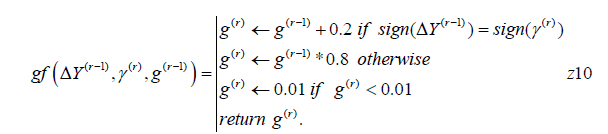The function takes as inputs the previous delta Y value, ΔY(r-1), the new gradient value, γ(r), and the previous gain value, g(r-1), and returns the new gain value, g(r). If the signs of the previous delta Y and new gradient values are the same, it increases the gain by 0.2; otherwise, it reduces the gain by 0.8. It also ensures that the new gain can never be less than 0.01.

The new gain is calculated as,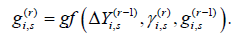The delta Y value,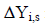is calculated as,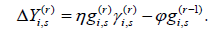The t-SNE algorithm sets η to 200 and the momentum constant, φ, to 0.5. In the first iteration, the Cen-se′ method finds the mean of the absolute values of the γ matrix elements and then sets η as,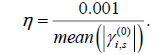The momentum constant, φ, is set to zero by default for the Cen-se′ method and will be examined in more detail in the Results Experiment 5 section.

The new Y values are calculated as,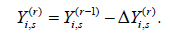The routine then returns to the Y. Normalization step and repeats the analysis a set number of iterations. The default number for t-SNE is 2000 and the default for Cen-se′ is 1000.

Citation: Bagwell CB, Bray CM, Herbert DJ, Hill BL, Inokuma MS, et al. (2019) Improving the t-SNE Algorithms for Cytometry and Other Technologies: Cen-Se′ Mapping. J Biom Biostat 10: 430.

Copyright: © 2019 Bagwell CB, et al. This is an open-access article distributed under the terms of the Creative Commons Attribution License, which permits unrestricted use, distribution, and reproduction in any medium, provided the original author and source are credited.

Select your language of interest to view the total content in your interested language

##### Recommended Journals
Viewmore
###### Article Usage
• Total views: 555
• [From(publication date): 0-0 - Nov 21, 2019]
• Breakdown by view type
• HTML page views: 512Can't read the image? click here to refresh